How Cheenta works to ensure student success?
Explore the Back-Story

# Length of the crease | AMC 10A, 2018 | Problem No 13Try this beautiful Problem on Geometry based on Length of the crease from AMC 10 A, 2018. You may use sequential hints to solve the problem.

## Length of the crease- AMC-10A, 2018- Problem 13

A paper triangle with sides of lengths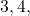and 5 inches, as shown, is folded so that pointfalls on point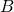. What is the length in inches of the crease?

,

••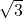••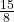•Geometry

Triangle

Pythagoras

## Suggested Book | Source | Answer

Pre College Mathematics

#### Source of the problem

AMC-10A, 2018 Problem-13

#### Check the answer here, but try the problem first## Try with Hints

#### First Hint

Given that ABC is a right-angle triangle shape paper. Now by the problem the pointmove on point. Therefore a crease will be create i.e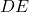. noe we have to find out the length of?

If you notice very carefully thenis the perpendicular bisector of the line. Therefore the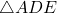is Right-angle triangle. Now the side lengths of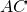,,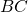are given. so if we can so that the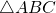then we can find out the side length of?

Now can you finish the problem?

#### Second Hint

Inandwe have ...( common angle)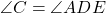(Right angle)

Therefore the remain angle will be equal ....

Therefore we can say thatNow Can you finish the Problem?

#### Third Hint

Astherefore we can write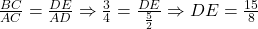Therefore the length in inches of the crease is## Subscribe to Cheenta at Youtube

Try this beautiful Problem on Geometry based on Length of the crease from AMC 10 A, 2018. You may use sequential hints to solve the problem.

## Length of the crease- AMC-10A, 2018- Problem 13

A paper triangle with sides of lengthsand 5 inches, as shown, is folded so that pointfalls on point. What is the length in inches of the crease?

,

•••••Geometry

Triangle

Pythagoras

## Suggested Book | Source | Answer

Pre College Mathematics

#### Source of the problem

AMC-10A, 2018 Problem-13

#### Check the answer here, but try the problem first## Try with Hints

#### First Hint

Given that ABC is a right-angle triangle shape paper. Now by the problem the pointmove on point. Therefore a crease will be create i.e. noe we have to find out the length of?

If you notice very carefully thenis the perpendicular bisector of the line. Therefore theis Right-angle triangle. Now the side lengths of,,are given. so if we can so that thethen we can find out the side length of?

Now can you finish the problem?

#### Second Hint

Inandwe have ...( common angle)(Right angle)

Therefore the remain angle will be equal ....

Therefore we can say thatNow Can you finish the Problem?

#### Third Hint

Astherefore we can writeTherefore the length in inches of the crease is## Subscribe to Cheenta at Youtube

This site uses Akismet to reduce spam. Learn how your comment data is processed.

### Knowledge Partner